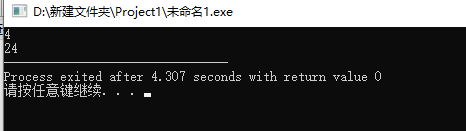2019-10-29 21:32

# 没有报错但是为什么没有结果 递归调用算阶乘

int main()
{
int jiecheng(int a);
int a;
scanf("%d", a);
printf("%d", jiecheng(a));
return 0;
}
int jiecheng( int a) {
float n, s = 0, t = 1;
for (n = 1; n <= a; n++) {
t *= n;
s += t;
}
return(s);
}

• 写回答
• 好问题 提建议
• 关注问题
• 收藏
• 邀请回答

#### 3条回答默认 最新

•threenewbee 2019-10-29 23:05
已采纳

递归是自己调用自己，也就是在jiecheng里又调用了jiecheng才是递归，正确写法如下，满意请采纳。

``````#include <stdio.h>

int main()
{
int jiecheng(int a);
int a;
scanf("%d", &a);
printf("%d", jiecheng(a));
return 0;
}
int jiecheng(int a)
{
if (a == 1) return 1;
return jiecheng(a - 1) * a;
}

``````
已采纳该答案
评论
解决 无用
打赏 举报
•chimpanzee 2019-10-29 22:35

首先你的代码没有递归调用
其次，看标题你是计算阶乘，n的阶乘=1x2x3x...x(n-1)xn；但是看你的jiecheng函数，返回值s计算的是小于等于n的所有正整数的阶乘的和，t才是n的阶乘；
最后，你说没有结果，可能跟你的变量定义有关，jiecheng函数定义返回值为int，但是你的s定义为float，工具及语言会不会自动转我也不太确定，你可以修改变量类型一致试一下

评论
解决 无用
打赏 举报
•_满天星_wjy 2019-10-29 22:35

#include
int main()
{
int jiecheng(int a);
int a;
scanf("%d", &a); //要用取地址符
printf("%d", jiecheng(a));
return 0;
}

int jiecheng( int a) {
float n, s = 0, t = 1;
for (n = 1; n <= a; n++)
{
t *= n;
s += t;
}
return(t); // T为阶乘值，S为N的阶乘值之和
}评论
解决 无用
打赏 举报